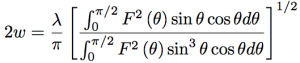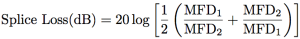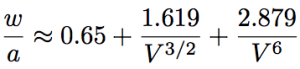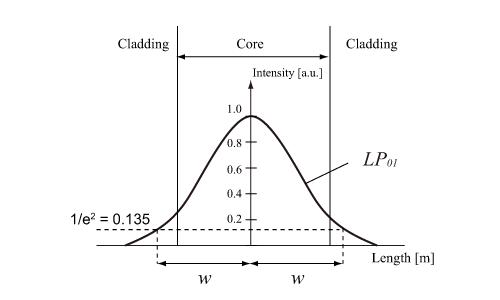In optical fiber, light propagates mainly through the core, but is partially leaked toward the clad. Especially for SMF, the diameter of the circular region for the light intensity of 1/e2=0.135 of the core center light intensity is defined as mode field diameter (provided that the light intensity distribution in SMF is approximated by the Gaussian function). MFD is schematically explained in Figure below. MFD, 2ω, is usually a little bit larger than the core diameter, therefore is important as an effective parameter representing spatial volume of the light propagation. The idea of MFD is yielded since the core diameter and relative refractive index difference in SMF are small, then the boundary between core and clad is hard to be validly identified. For typical SMFs, MFD is in the range of 3~10 µm.

According to the ITU-T advisory, MFD is defined by the following equation,where F(θ) is the electric field distribution of far field pattern (FFP) and θ is the radiation angle to the propagation axis of optical fiber. MFD is also employed for evaluating the fiber splicing loss. The splice loss and MFD has the relationship shown below.In above equation, MFD1 and MFD2 are MFD of a couple of fibers for splicing.

For SMF, the ratio of w to the core radius, a (w/a), is represented by the following function of V parameter.Above equation indicates that MFD is relatively smaller for the larger V parameter. The equation can be used for the limited values of V parameter, 0.8~2.5.Fig. Schematic of mode field diameter.